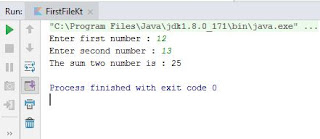# Program to add two numbers in kotlin

In this article, we will share an example of Kotlin program to add two numbers. Further, you will learn to store the value in a variable and then sum two variables. Let's see how to write a program to add two integer number in Kotlin.

Source Code:
fun main(args: Array<String>) {

print("Enter first number : ")
print("Enter second number : ")

if(firstnumber.toIntOrNull() != null && secondnumber.toIntOrNull() != null)
{
val sum: Int = firstnumber.toInt() + secondnumber.toInt()
println("The sum two number is : \$sum")
}
else
{
println("Please enter valid first and second number.")
}
}

Output:
Enter first number : 12
Enter second number : 13
The sum two number is : 25Kotlin program to add two numbers - output

Description:
In this program, we have taken two variable "firstnumber" and "secondnumber", in which we are taking input from the end user. To do so we are using readLine()!! function.

After taking two number from the user, we have checked that entered text is number or not. To do so, we have used toIntOrNull() function. if the text is not integer number, it will return null value.

If it does satisfy the condition and entered text are integer number, then it will add two number using "+" operator. Next, it will print the same using print() function.

If it does not satisfy the condition, it will go to else statement and print the message to enter valid numbers.